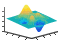# fsurf

•## 语法

fsurf(f)
fsurf(f,xyinterval)
fsurf(funx,funy,funz)
fsurf(funx,funy,funz,uvinterval)
fsurf(___,LineSpec)
fsurf(___,Name,Value)
fsurf(ax,___)
fs = fsurf(___)

## 说明

fsurf(f) 在默认区间 [-5 5]（对于 x 和 y）为函数 z = f(x,y) 创建曲面图。
fsurf(f,xyinterval) 将在指定区间绘图。要对 x 和 y 使用相同的区间，请将 xyinterval 指定为 [min max] 形式的二元素向量。要使用不同的区间，请指定 [xmin xmax ymin ymax] 形式的四元素向量。

fsurf(funx,funy,funz) 在默认区间 [-5 5]（对于 u 和 v）绘制由 x = funx(u,v)、y = funy(u,v)、z = funz(u,v) 定义的参数化曲面。
fsurf(funx,funy,funz,uvinterval) 将在指定区间绘图。要对 u 和 v 使用相同的区间，请将 uvinterval 指定为 [min max] 形式的二元素向量。要使用不同的区间，请指定 [umin umax vmin vmax] 形式的四元素向量。
fsurf(___,LineSpec) 设置线型、标记符号和曲面颜色。例如，'-r' 指定红色线条。在前面的任何输入参数组合之后使用此选项。

fsurf(___,Name,Value) 使用一个或多个名称-值对组参数指定曲面属性。在上述语法中的任何输入参数组合后使用此选项。
fsurf(ax,___) 将图形绘制到 ax 指定的坐标区而不是当前坐标区 (gca) 中。

fs = fsurf(___) 返回 FunctionSurface 对象或 ParameterizedFunctionSurface 对象，具体情况取决于输入。使用 fs 来查询和修改特定曲面的属性。有关属性列表，请参阅 FunctionSurface 属性 或 ParameterizedFunctionSurface 属性。

## 示例

fsurf(@(x,y) sin(x)+cos(y))$\begin{array}{cc}erf\left(x\right)+\mathrm{cos}\left(y\right)& -5

f1 = @(x,y) erf(x)+cos(y); fsurf(f1,[-5 0 -5 5]) hold on f2 = @(x,y) sin(x)+cos(y); fsurf(f2,[0 5 -5 5]) hold off$\begin{array}{c}x=r\mathrm{cos}\left(u\right)\mathrm{sin}\left(v\right)\\ y=r\mathrm{sin}\left(u\right)\mathrm{sin}\left(v\right)\\ z=r\mathrm{cos}\left(v\right)\\ where\phantom{\rule{1em}{0ex}}r=2+\mathrm{sin}\left(7u+5v\right)\end{array}$

r = @(u,v) 2 + sin(7.*u + 5.*v); funx = @(u,v) r(u,v).*cos(u).*sin(v); funy = @(u,v) r(u,v).*sin(u).*sin(v); funz = @(u,v) r(u,v).*cos(v); fsurf(funx,funy,funz,[0 2*pi 0 pi]) camlightfsurf(@(x,y) y.*sin(x)-x.*cos(y),[-2*pi 2*pi]) title('ysin(x) - xcos(y) for x and y in [-2\pi,2\pi]') xlabel('x'); ylabel('y'); zlabel('z'); box onax = gca; ax.XTick = -2*pi:pi/2:2*pi; ax.XTickLabel = {'-2\pi','-3\pi/2','-\pi','-\pi/2','0',... '\pi/2','\pi','3\pi/2','2\pi'}; ax.YTick = -2*pi:pi/2:2*pi; ax.YTickLabel = {'-2\pi','-3\pi/2','-\pi','-\pi/2','0',... '\pi/2','\pi','3\pi/2','2\pi'};funx = @(u,v) u.*sin(v); funy = @(u,v) -u.*cos(v); funz = @(u,v) v; fsurf(funx,funy,funz,[-5 5 -5 -2],'--','EdgeColor','g') hold on fsurf(funx,funy,funz,[-5 5 -2 2],'EdgeColor','none') hold off$\begin{array}{c}x={e}^{-|u|/10}\mathrm{sin}\left(5|v|\right)\\ y={e}^{-|u|/10}\mathrm{cos}\left(5|v|\right)\\ z=u.\end{array}$

x = @(u,v) exp(-abs(u)/10).*sin(5*abs(v)); y = @(u,v) exp(-abs(u)/10).*cos(5*abs(v)); z = @(u,v) u; fs = fsurf(x,y,z)fs = ParameterizedFunctionSurface with properties: XFunction: @(u,v)exp(-abs(u)/10).*sin(5*abs(v)) YFunction: @(u,v)exp(-abs(u)/10).*cos(5*abs(v)) ZFunction: @(u,v)u EdgeColor: [0 0 0] LineStyle: '-' FaceColor: 'interp' Show all properties 

fs.URange = [-30 30]; fs.FaceAlpha = .5;f = @(x,y) 3*(1-x).^2.*exp(-(x.^2)-(y+1).^2)... - 10*(x/5 - x.^3 - y.^5).*exp(-x.^2-y.^2)... - 1/3*exp(-(x+1).^2 - y.^2); fsurf(f,[-3 3],'ShowContours','on')tiledlayout(2,1) nexttile fsurf(@(s,t) sin(s), @(s,t) cos(s), @(s,t) t/10.*sin(1./s)) view(-172,25) title('Default MeshDensity = 35') nexttile fsurf(@(s,t) sin(s), @(s,t) cos(s),... @(s,t) t/10.*sin(1./s),'MeshDensity',40) view(-172,25) title('Increased MeshDensity = 40')## 输入参数

xy 的绘图区间，指定为下列形式之一：

• [min max] 形式的向量 - 对 xy 都使用区间 [min max]

• [xmin xmax ymin ymax] 形式的向量 - 对 x 使用区间 [xmin xmax]，对 y 使用区间 [ymin ymax]

x 坐标的参数化函数，指定为命名或匿名函数的函数句柄。

y 坐标的参数化函数，指定为命名或匿名函数的函数句柄。

z 坐标的参数化函数，指定为命名或匿名函数的函数句柄。

uv 的绘图区间，指定为下列形式之一：

• [min max] 形式的向量 - 对 uv 都使用区间 [min max]

• [umin umax vmin vmax] 形式的向量 - 对 u 使用区间 [umin umax]，对 v 使用区间 [vmin vmax]

"-"实线"--"虚线":"点线"-."点划线"o"圆圈"+"加号"*"星号".""x"叉号"_"水平线条"|"垂直线条"square"方形"diamond"菱形"^"上三角"v"下三角">"右三角"<"左三角"pentagram"五角形"hexagram"六角形"red""r"[1 0 0]"green""g"[0 1 0]"blue""b"[0 0 1]"cyan" "c"[0 1 1]"magenta""m"[1 0 1]"yellow""y"[1 1 0]"black""k"[0 0 0]"white""w"[1 1 1]### 名称-值参数

• RGB 三元组是包含三个元素的行向量，其元素分别指定颜色中红、绿、蓝分量的强度。强度值必须位于 [0,1] 范围内，例如 [0.4 0.6 0.7]

• 十六进制颜色代码是字符串标量或字符向量，以井号 (#) 开头，后跟三个或六个十六进制数字，范围可以是 0F。这些值不区分大小写。因此，颜色代码 "#FF8800""#ff8800""#F80""#f80" 是等效的。

"red""r"[1 0 0]"#FF0000""green""g"[0 1 0]"#00FF00""blue""b"[0 0 1]"#0000FF""cyan" "c"[0 1 1]"#00FFFF""magenta""m"[1 0 1]"#FF00FF""yellow""y"[1 1 0]"#FFFF00""black""k"[0 0 0]"#000000""white""w"[1 1 1]"#FFFFFF""none"不适用不适用不适用无颜色

RGB 三元组十六进制颜色代码外观
[0 0.4470 0.7410]"#0072BD"[0.8500 0.3250 0.0980]"#D95319"[0.9290 0.6940 0.1250]"#EDB120"[0.4940 0.1840 0.5560]"#7E2F8E"[0.4660 0.6740 0.1880]"#77AC30"[0.3010 0.7450 0.9330]"#4DBEEE"[0.6350 0.0780 0.1840]"#A2142F""-"实线"--"虚线":"点线"-."点划线"none"无线条无线条

"o"圆圈"+"加号"*"星号".""x"叉号"_"水平线条"|"垂直线条"square"方形"diamond"菱形"^"上三角"v"下三角">"右三角"<"左三角"pentagram"五角形"hexagram"六角形"none"无标记不适用

## 输出参数

• 如果使用 fsurf(f) 语法或此语法的变体，fsurf 将返回 FunctionSurface 对象。

• 如果使用 fsurf(funx,funy,funz) 语法或此语法的变体，fsurf 将返回 ParameterizedFunctionSurface 对象。